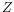# Structure Scanning Plot

In CLC Genomics Workbench it is possible to scan larger sequences for the existence of local conserved RNA structures. The structure scanning approach is similar in spirit to the works of [Workman and Krogh, 1999] and [Clote et al., 2005]. The idea is that if natural selection is operating to maintain a stable local structure in a given region, then the minimum free energy of the region will be markedly lower than the minimum free energy found when the nucleotides of the subsequence are distributed in random order.

The algorithm works by sliding a window along the sequence. Within the window, the minimum free energy of the subsequence is calculated. To evaluate the significance of the local structure signal its minimum free energy is compared to a background distribution of minimum free energies obtained from shuffled sequences, using-scores [Rivas and Eddy, 2000]. The-score statistics corresponds to the number of standard deviations by which the minimum free energy of the original sequence deviates from the average energy of the shuffled sequences. For a given-score, the statistical significance is evaluated as the probability of observing a more extreme-score under the assumption that-scores are normally distributed [Rivas and Eddy, 2000].

Subsections